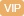| APP | 校园号 | 客服 客服热线：400-863-9889

400-863-9889（1）爷爷用放大镜看报．

（2）王老师用投影仪投放幻灯片．

（3）李叔叔给校园画平面图．

【考点】图形的放大与缩小【专题】图形与变换．

【分析】先计算小号里面的，后从向右依次计即可；

 13 49
×2+13×
 28 49

 13 49
×1+28×
 13 49
，然后据乘分配律简算可．
【解答】解：（
 4 5
+
 1 4
）÷
 7 3
+
 7 10

=
 13 49
×（2+28）
=1
 3 20

=
 21 20
×
 3 7
+
 7 10

=.6
=7.6×.5+065×7.6
=
 13 49
×21+2×
 13 49

=7.6（.35+0.6）
=96
7.2-3.4+1.72-86
 13 49
21+13×
 28 49

=76×1
=3
=13
【点评】题主考查了分的简便运算，要熟，意乘法运算定律加法运算定律的应用．

0/0